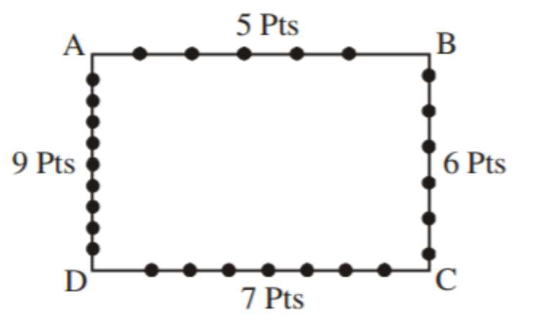# Consider a rectangle ABCD havingQuestion:

Consider a rectangle $\mathrm{ABCD}$ having $5,7,6,9$ points in the interior of the line segments $\mathrm{AB}, \mathrm{CD}, \mathrm{BC}, \mathrm{DA}$ respectively. Let $\alpha$ be the numberof triangles having these points from different sides as vertices and $\beta$ be the number of quadrilaterals having these points from different sides as vertices. Then $(\beta-\alpha)$ is equal to :

1. (1) 795

2. (2) 1173

3. (3) 1890

4. (4) 717

Correct Option: , 4

Solution:$\alpha=$ Number of triangles

$\alpha=5 \cdot 6 \cdot 7+5 \cdot 7 \cdot 9+5 \cdot 6 \cdot 9+6 \cdot 7 \cdot 9$

$=210+315+270+378$

$=1173$

$\beta=$ Number of Quadrilateral

$\beta=5 \cdot 6 \cdot 7 \cdot 9=1890$

$\beta-\alpha=1890-1173=717$